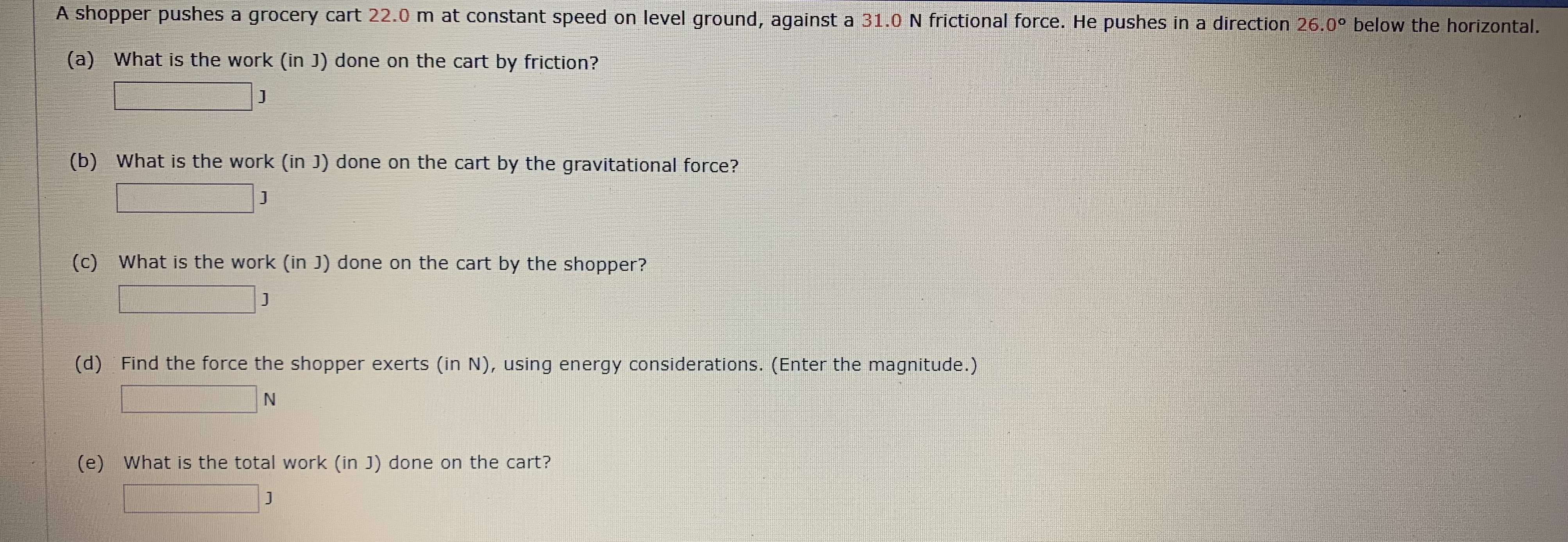# A shopper pushes a grocery cart 22.0 m at constant speed on level ground, against a 31.0 N frictional force. He pushes in a direction 26.00 below the horizontal. (a) What is the work (in J) done on the cart by friction? J (b) What is the work (in J) done on the cart by the gravitational force? J (c) What is the work (in J) done on the cart by the shopper? J Find the force the shopper exerts (in N), using energy considerations. (Enter the magnitude.) (d) N What is the total work (in J) done on the cart? (e) J

Questionhelp_outlineImage TranscriptioncloseA shopper pushes a grocery cart 22.0 m at constant speed on level ground, against a 31.0 N frictional force. He pushes in a direction 26.00 below the horizontal. (a) What is the work (in J) done on the cart by friction? J (b) What is the work (in J) done on the cart by the gravitational force? J (c) What is the work (in J) done on the cart by the shopper? J Find the force the shopper exerts (in N), using energy considerations. (Enter the magnitude.) (d) N What is the total work (in J) done on the cart? (e) J fullscreen

### Want to see the step-by-step answer?